Next: Time-Independent Perturbation Theory Up: Two Spin One-Half Particles Previous: Two Spin One-Half Particles

### Exercises

1. An electron in a hydrogen atom occupies the combined spin and position state1. What values would a measurement of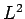yield, and with what probabilities?
2. Same for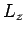.
3. Same for.
4. Same for.
5. Same for.
6. Same for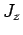.
7. What is the probability density for finding the electron at,,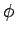?
8. What is the probability density for finding the electron in the spin up state (with respect to the-axis) at radius?

2. In a low energy neutron-proton system (with zero orbital angular momentum) the potential energy is given bywhere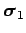denotes the vector of the Pauli matrices of the neutron, and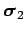denotes the vector of the Pauli matrices of the proton. Calculate the potential energy for the neutron-proton system:
1. In the spin singlet state.
2. In the spin triplet state.

3. Consider two electrons in a spin singlet state.
1. If a measurement of the spin of one of the electrons shows that it is in the state with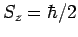, what is the probability that a measurement of the-component of the spin of the other electron yields?
2. If a measurement of the spin of one of the electrons shows that it is in the state with, what is the probability that a measurement of the-component of the spin of the other electron yields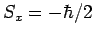?
Finally, if electron 1 is in a spin state described by, and electron 2 is in a spin state described by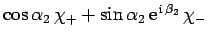, what is the probability that the two-electron spin state is a triplet state?Next: Time-Independent Perturbation Theory Up: Two Spin One-Half Particles Previous: Two Spin One-Half Particles
Richard Fitzpatrick 2010-07-20